# ggthemes Package in R (Example)

In this R programming tutorial you’ll learn how to modify the theme of a ggplot2 plot using the ggthemes package.

The tutorial consists of one example for the modification of the theme of a ggplot2 plot using the ggthemes package. To be more specific, the content of the article looks as follows:

Let’s get started.

## Example Data, Packages & Default Graph

The first step is to create some data that we can use in the examples below:

```set.seed(89365343)                               # Set random seed
data <- data.frame(x = rnorm(100),               # Create example data frame
y = rnorm(100),
group = LETTERS[1:5])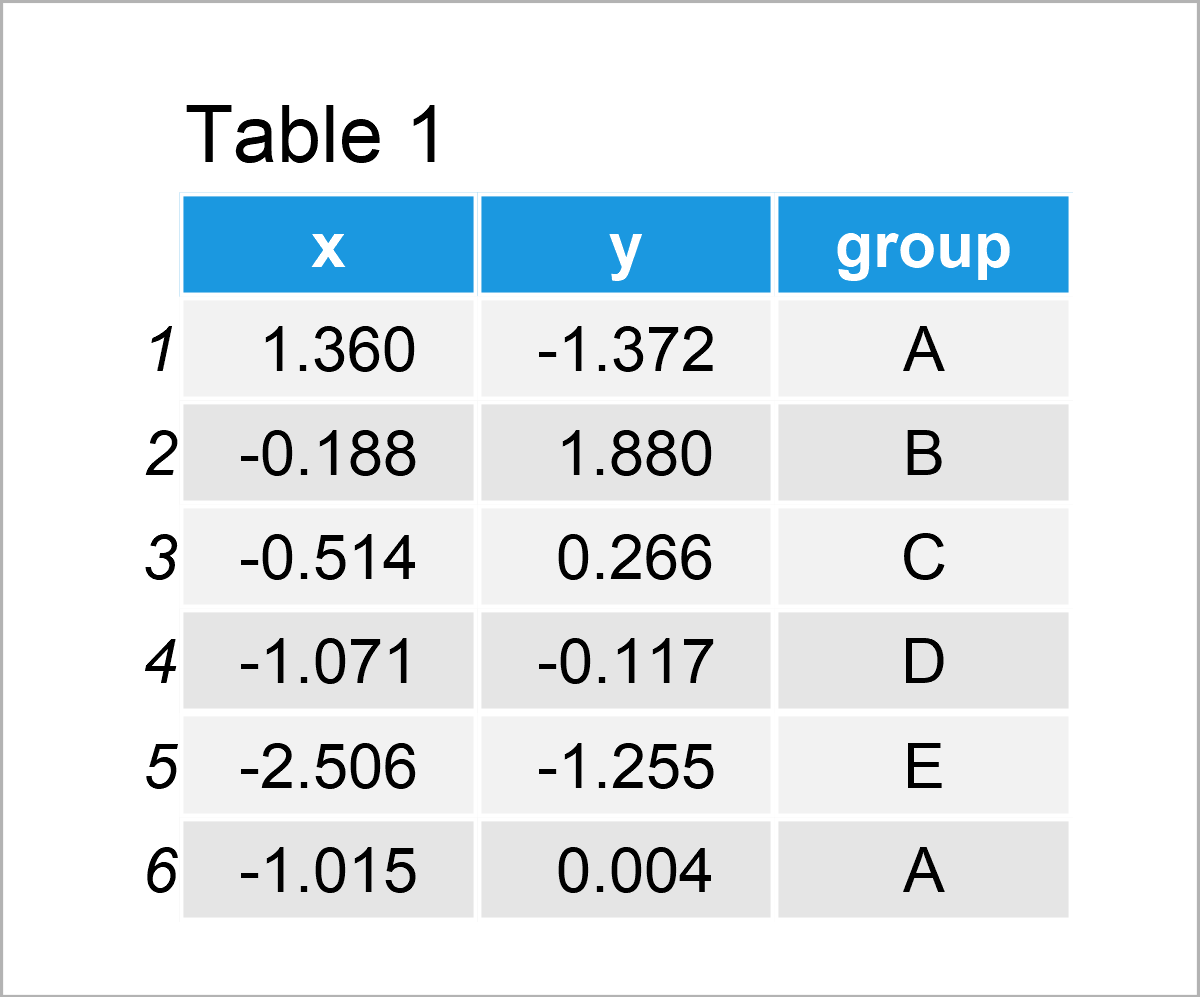Table 1 shows that our example data has three columns. The variables x and y have the numeric class and the variable group is a character.

For the following tutorial, we also need to install and load the ggplot2 software package:

```install.packages("ggplot2")                      # Install ggplot2 package

As a next step, we can plot our data with default theme specifications, i.e. the theme_gray theme of the ggplot2 package:

```ggp <- ggplot(data, aes(x, y, col = group)) +    # Draw default ggplot2 plot
geom_point()
ggp```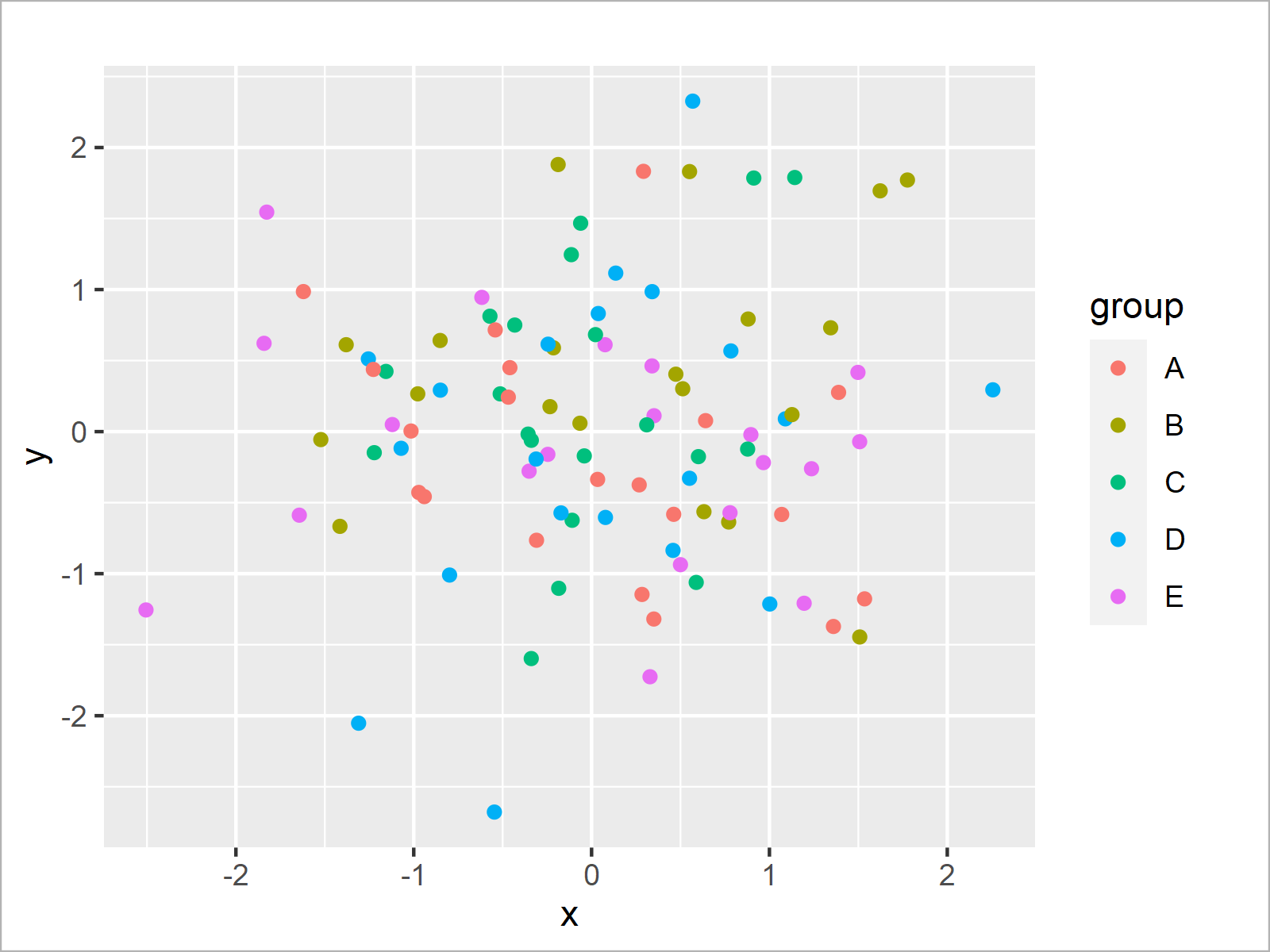After executing the previous syntax the default ggplot2 scatterplot shown in Figure 1 has been created.

## Example: Draw ggplot2 Plot Using Themes of ggthemes Package

This section demonstrates how to change the theme of a ggplot2 graph using the functions of the ggthemes package.

If we want to use the functions of the ggthemes package, we first need to download, install and load ggthemes. Otherwise, the error message there is no package called ggthemes would occur:

```install.packages("ggthemes")                     # Install ggthemes package

The ggthemes package provides extra themes, geoms, and scales for the ggplot2 package. In this tutorial, we’ll focus on the additional themes that are provided by ggthemes.

In the next step, we can add the theme that we want to use to our ggplot2 plot object called ggp that we have created in the previous section of this tutorial.

The following R codes and their graphical outputs show the different themes that are provided by the ggthemes package.

```ggp_base <- ggp + theme_base() + ggtitle("theme_base()")
ggp_base``````ggp_calc <- ggp + theme_calc() + ggtitle("theme_calc()")
ggp_calc``````ggp_clean <- ggp + theme_clean() + ggtitle("theme_clean()")
ggp_clean```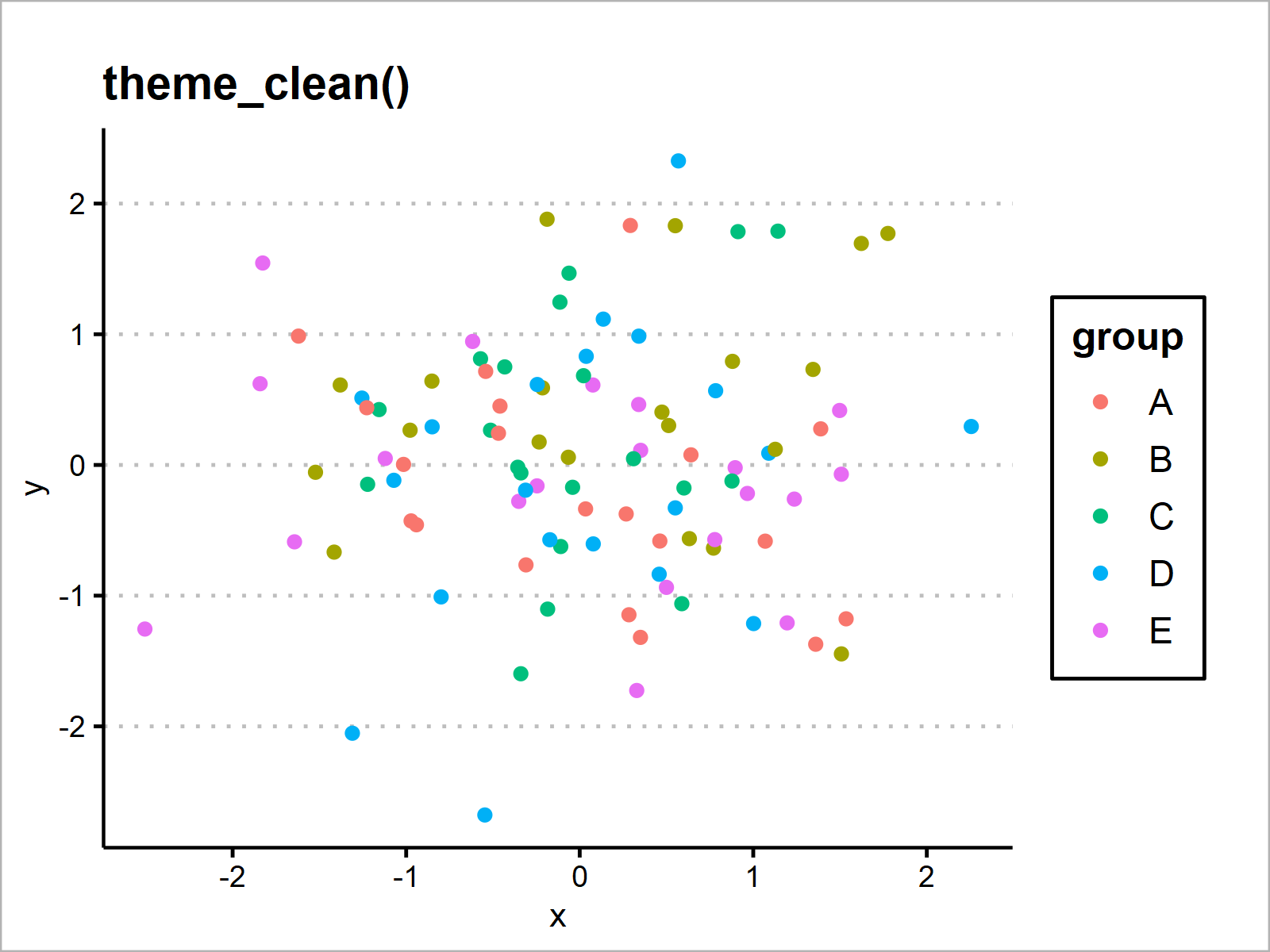```ggp_economist <- ggp + theme_economist() + ggtitle("theme_economist()")
ggp_economist```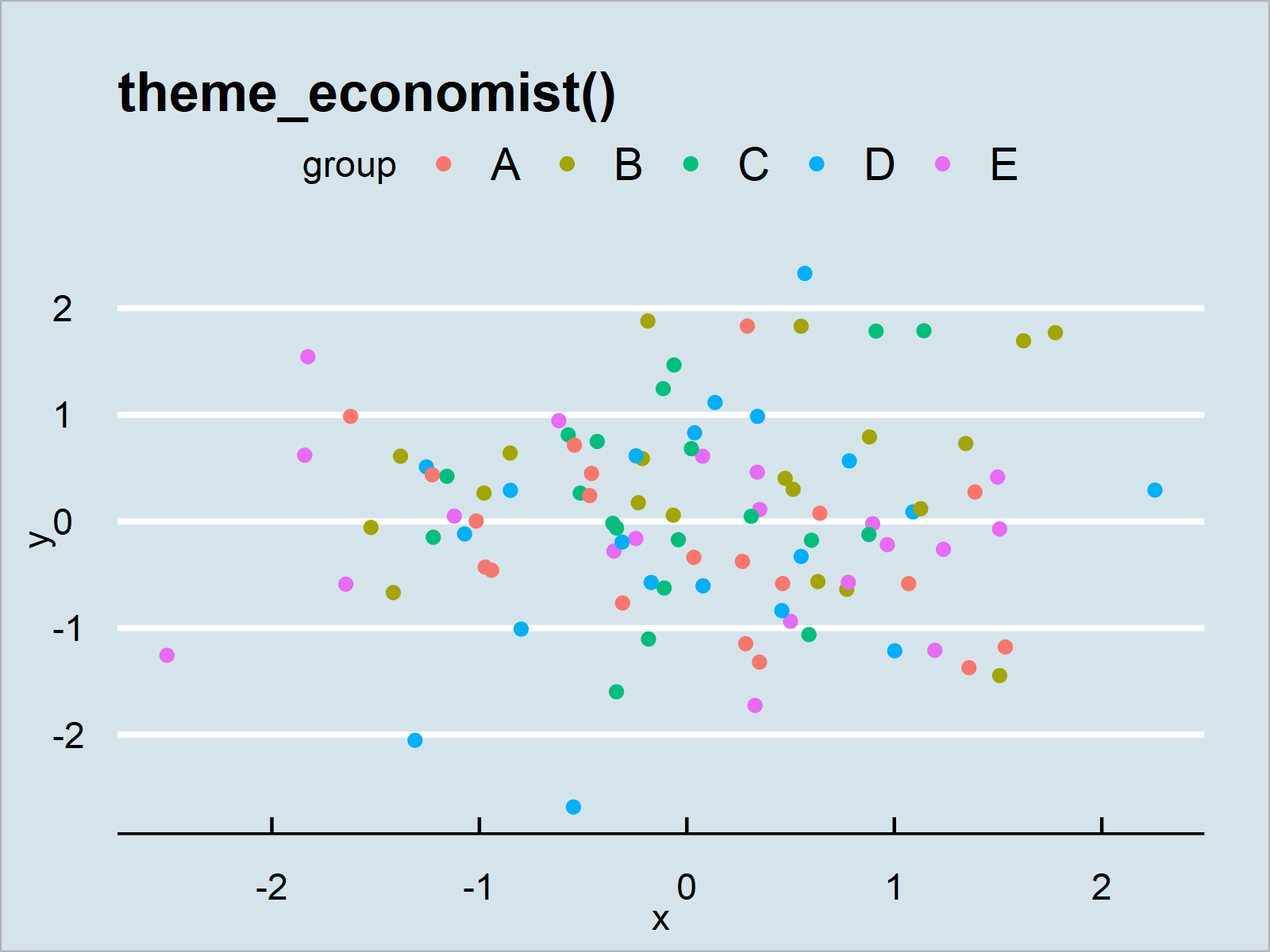```ggp_economist_white <- ggp + theme_economist_white() + ggtitle("theme_economist_white()")
ggp_economist_white```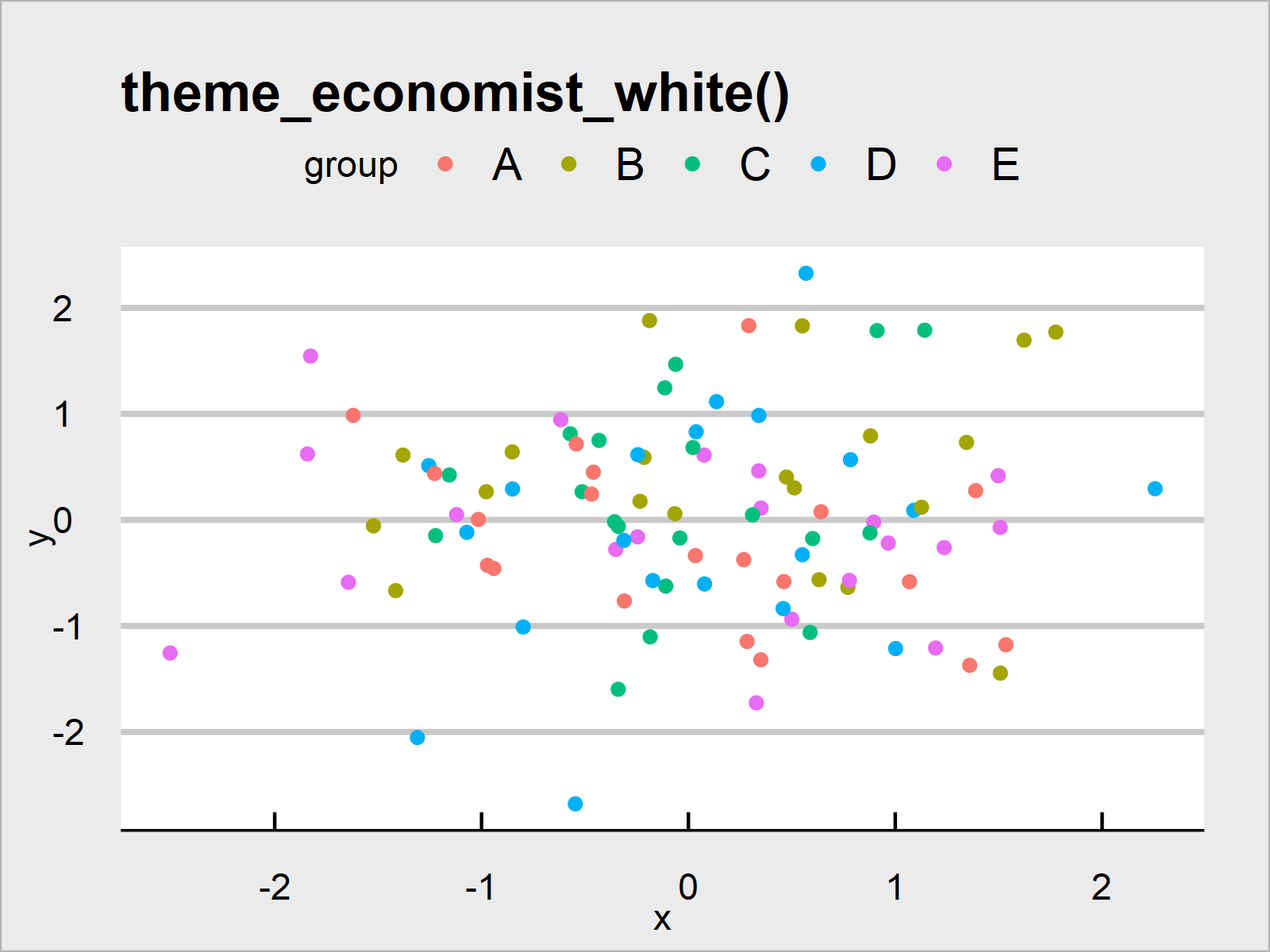```ggp_excel <- ggp + theme_excel() + ggtitle("theme_excel()")
ggp_excel``````ggp_excel_new <- ggp + theme_excel_new() + ggtitle("theme_excel_new()")
ggp_excel_new```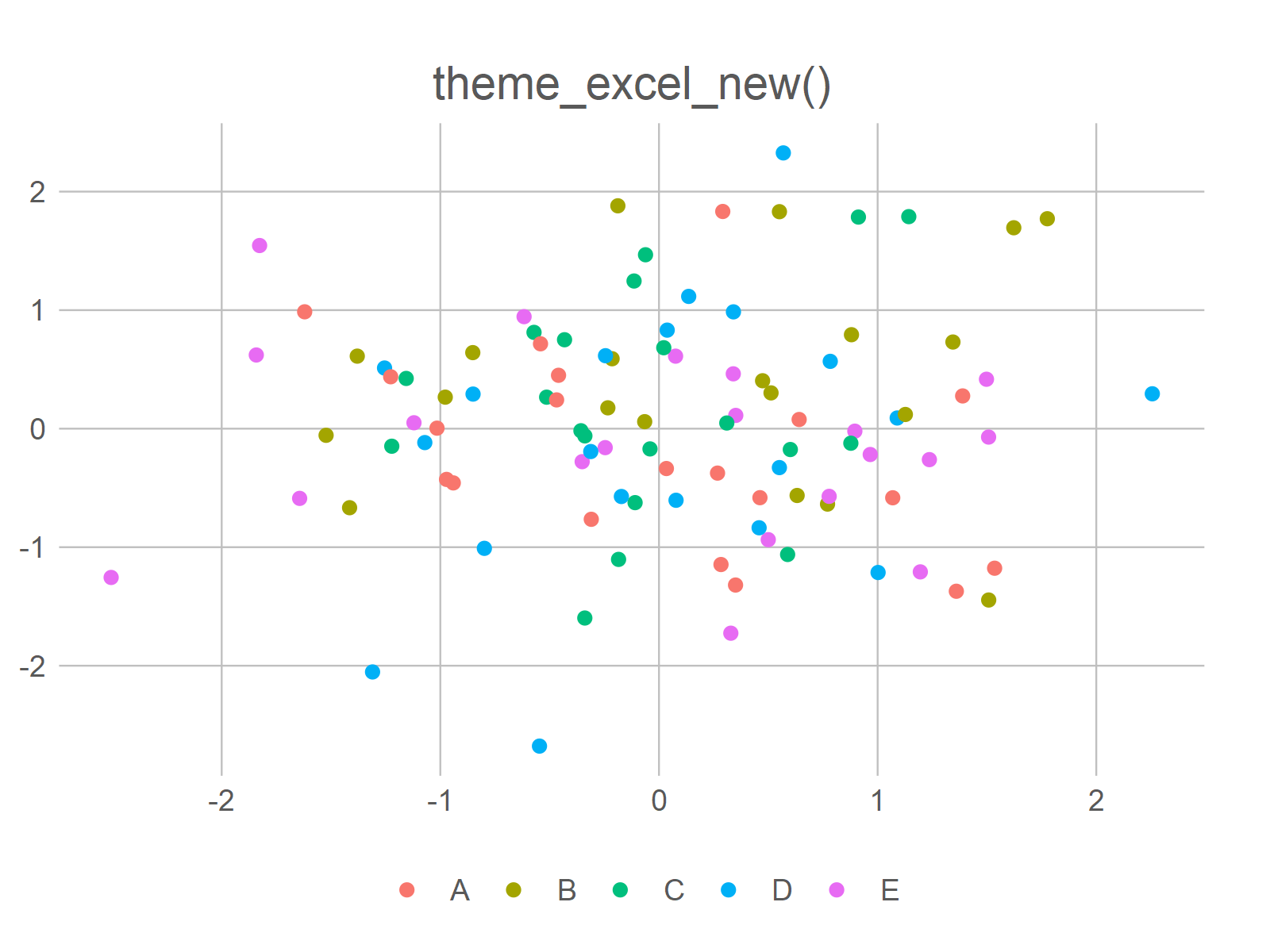```ggp_few <- ggp + theme_few() + ggtitle("theme_few()")
ggp_few```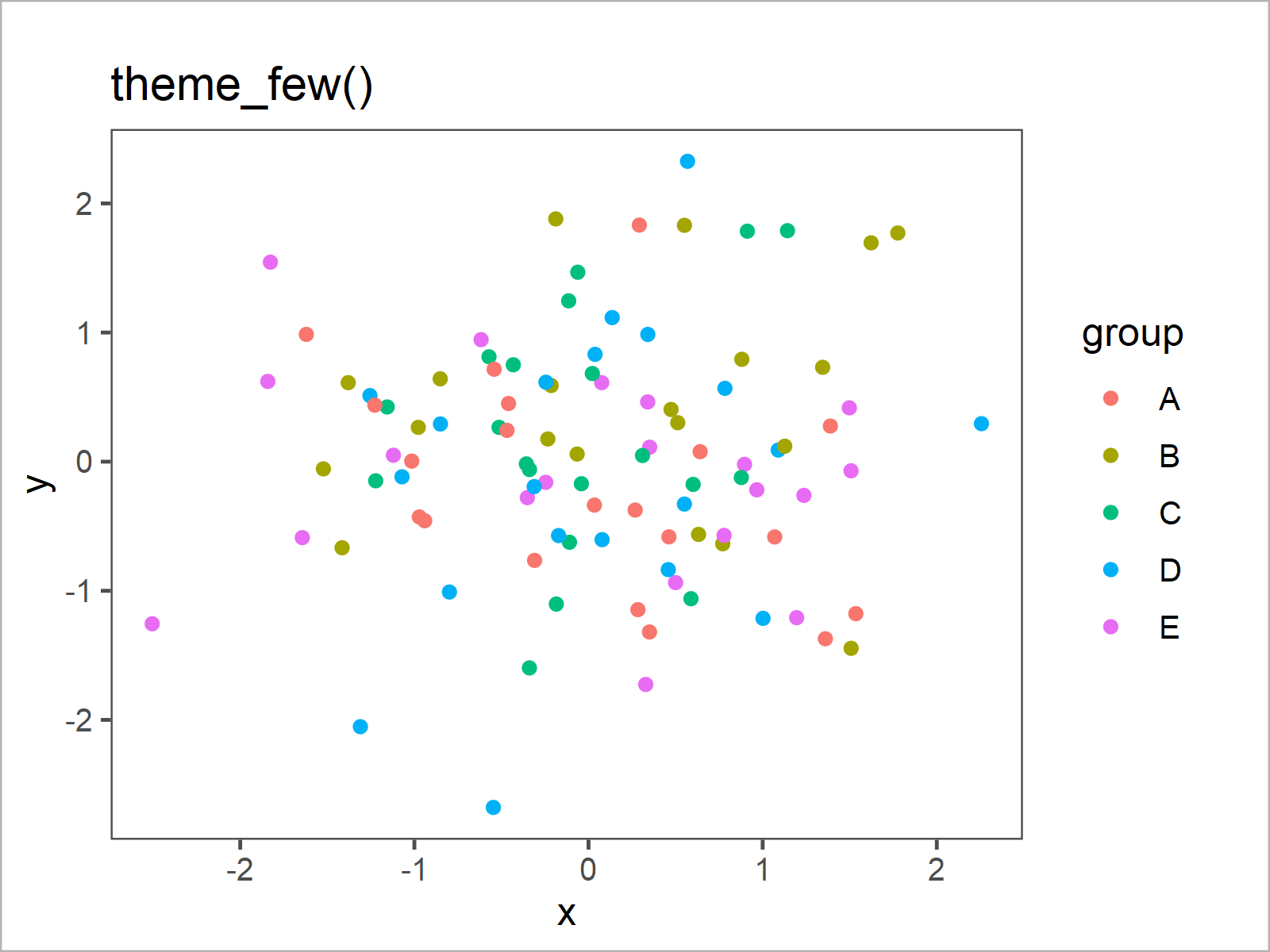```ggp_fivethirtyeight <- ggp + theme_fivethirtyeight() + ggtitle("theme_fivethirtyeight()")
ggp_fivethirtyeight```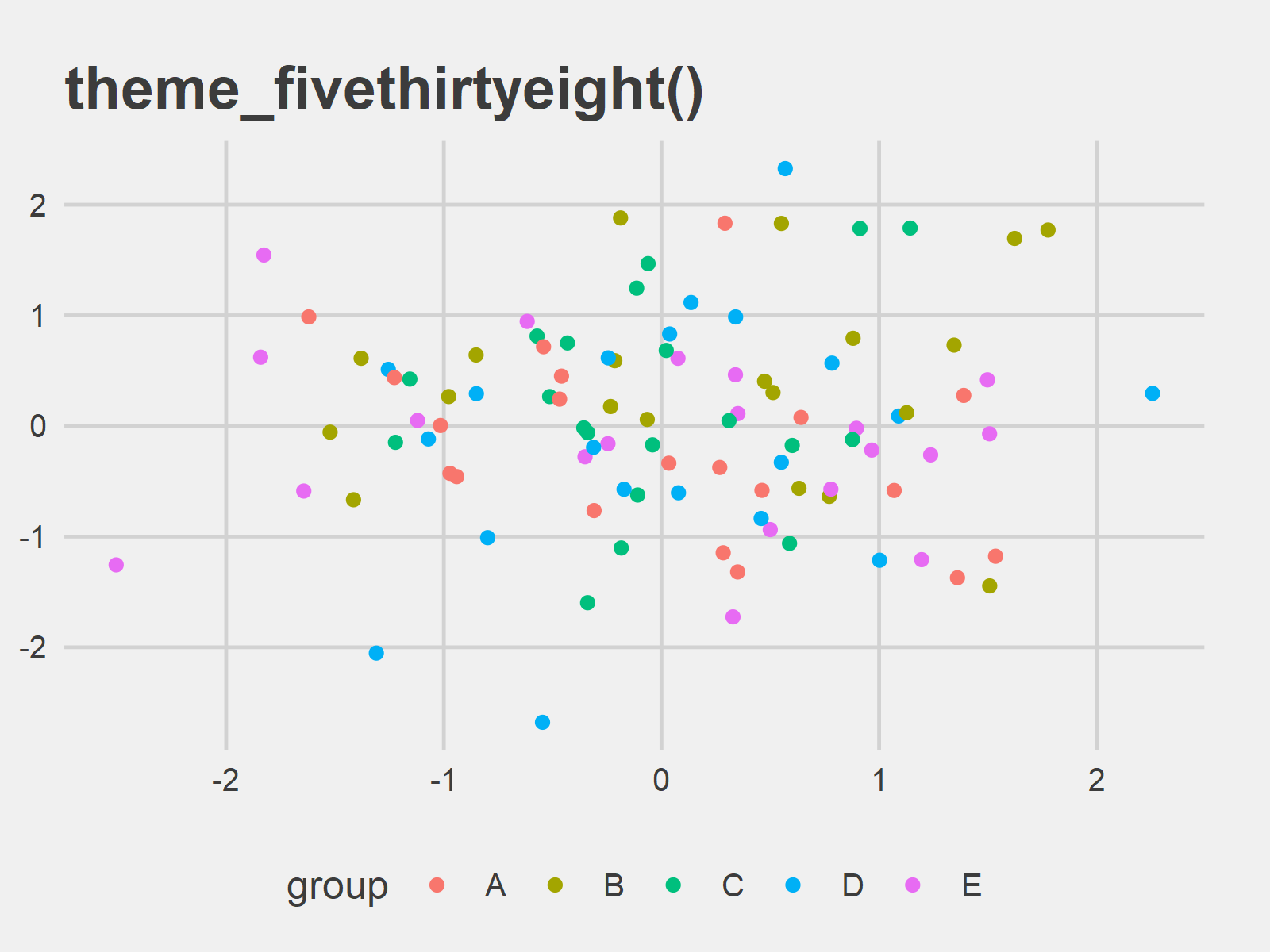```ggp_foundation <- ggp + theme_foundation() + ggtitle("theme_foundation()")
ggp_foundation``````ggp_gdocs <- ggp + theme_gdocs() + ggtitle("theme_gdocs()")
ggp_gdocs```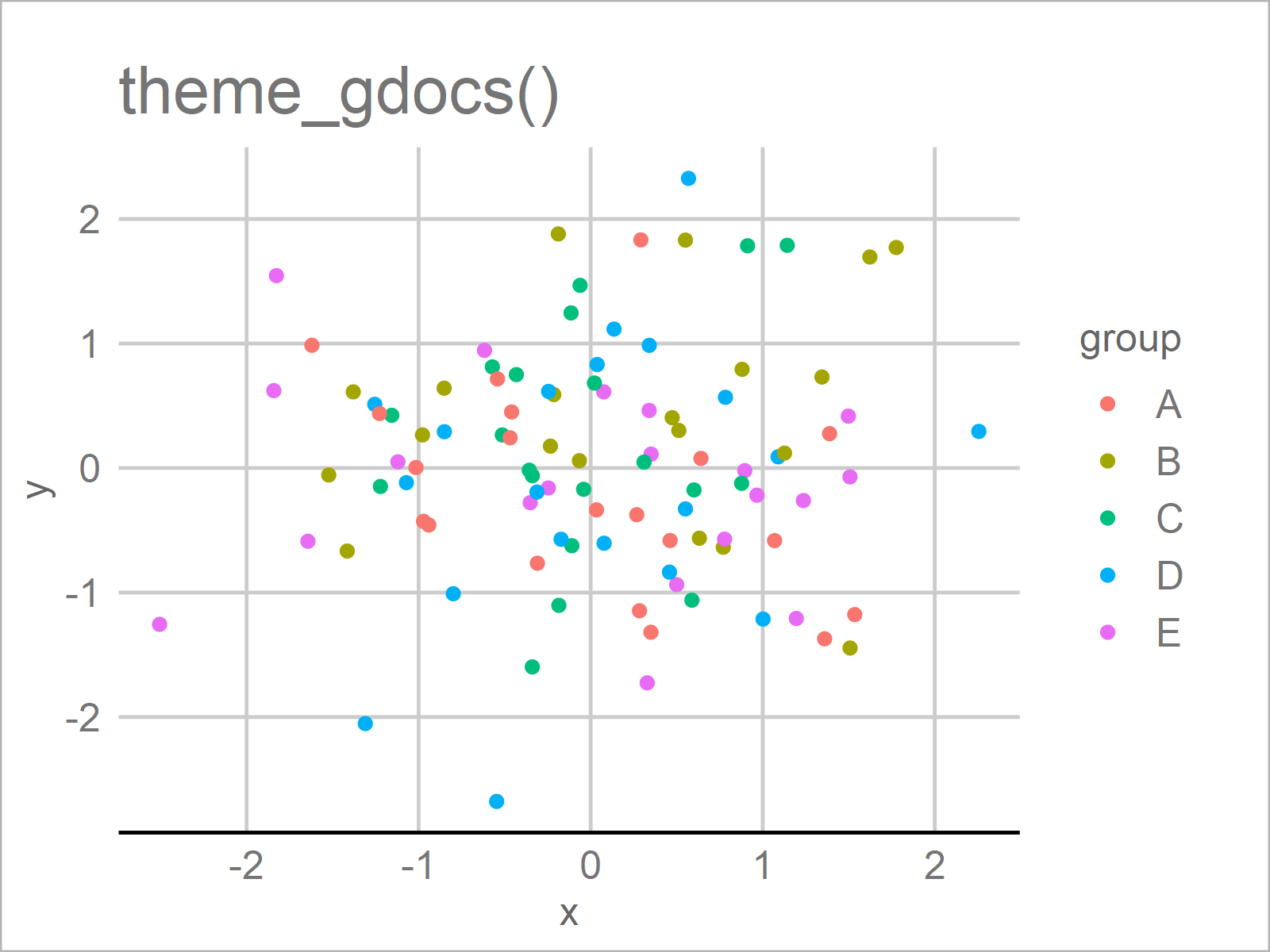```ggp_hc <- ggp + theme_hc() + ggtitle("theme_hc()")
ggp_hc``````ggp_igray <- ggp + theme_igray() + ggtitle("theme_igray()")
ggp_igray```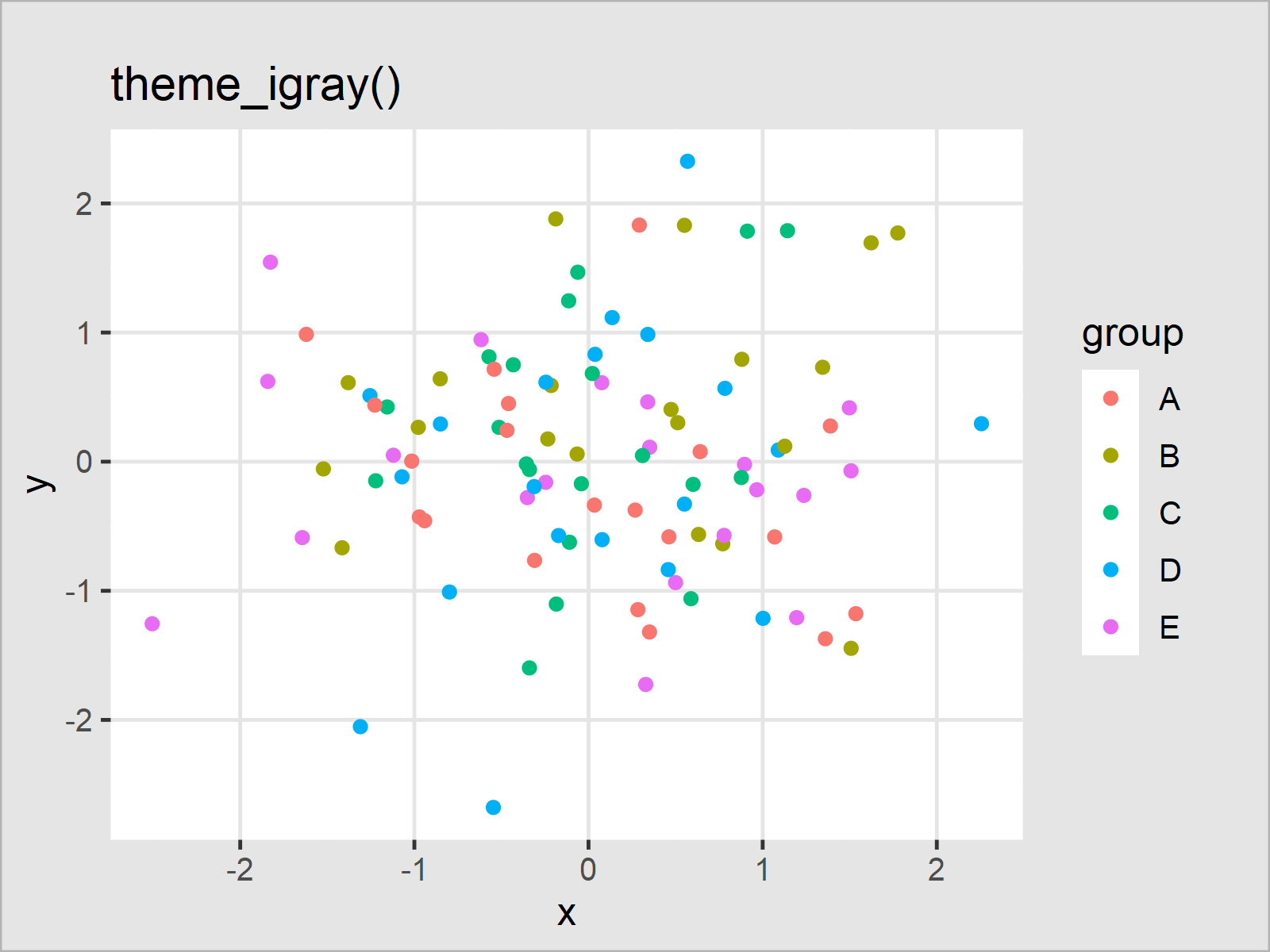```ggp_map <- ggp + theme_map() + ggtitle("theme_map()")
ggp_map```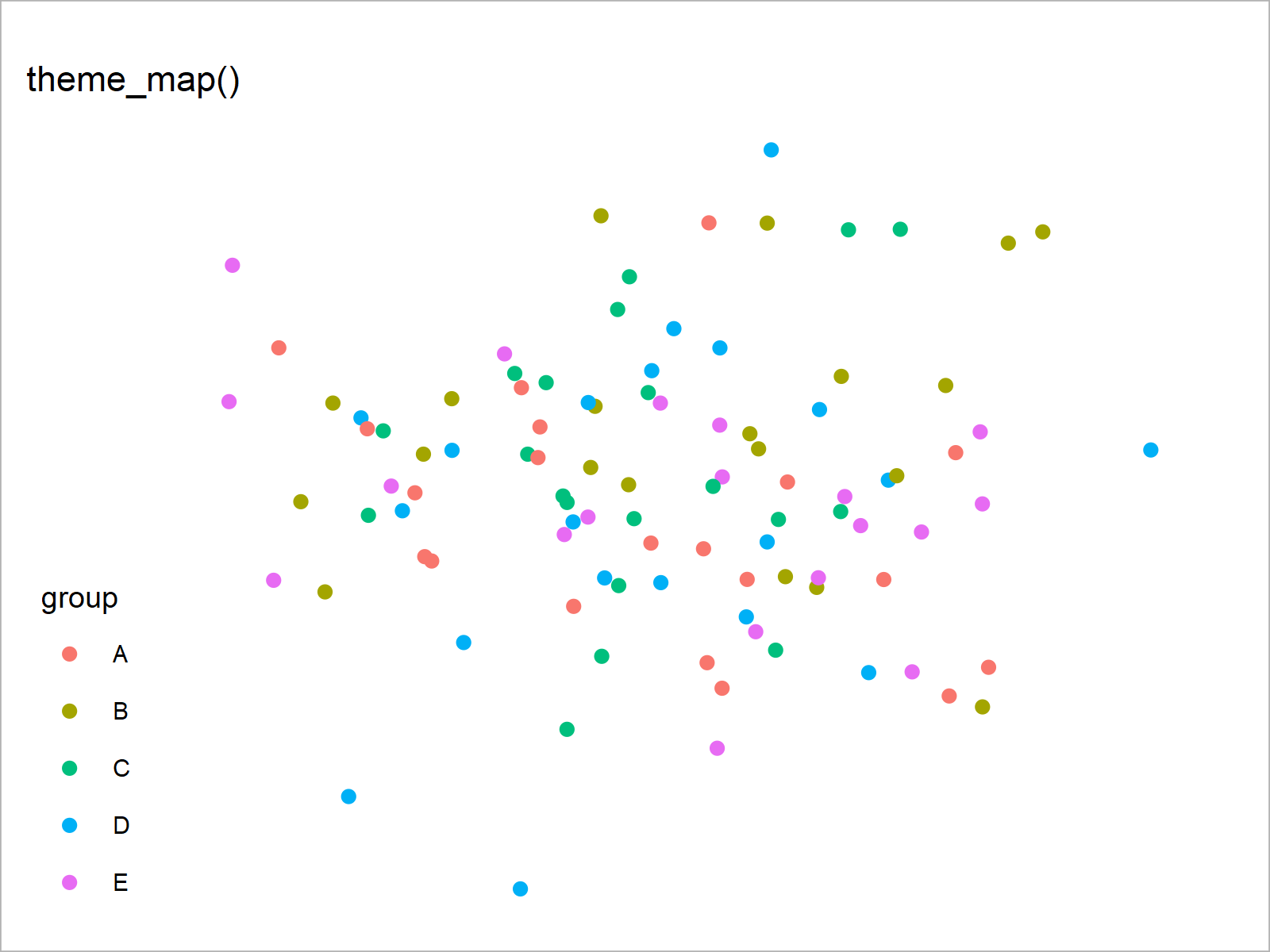```ggp_pander <- ggp + theme_pander() + ggtitle("theme_pander()")
ggp_pander``````ggp_par <- ggp + theme_par() + ggtitle("theme_par()")
ggp_par``````ggp_solarized <- ggp + theme_solarized() + ggtitle("theme_solarized()")
ggp_solarized``````ggp_solarized_2 <- ggp + theme_solarized_2() + ggtitle("theme_solarized_2()")
ggp_solarized_2``````ggp_solid <- ggp + theme_solid() + ggtitle("theme_solid()")
ggp_solid```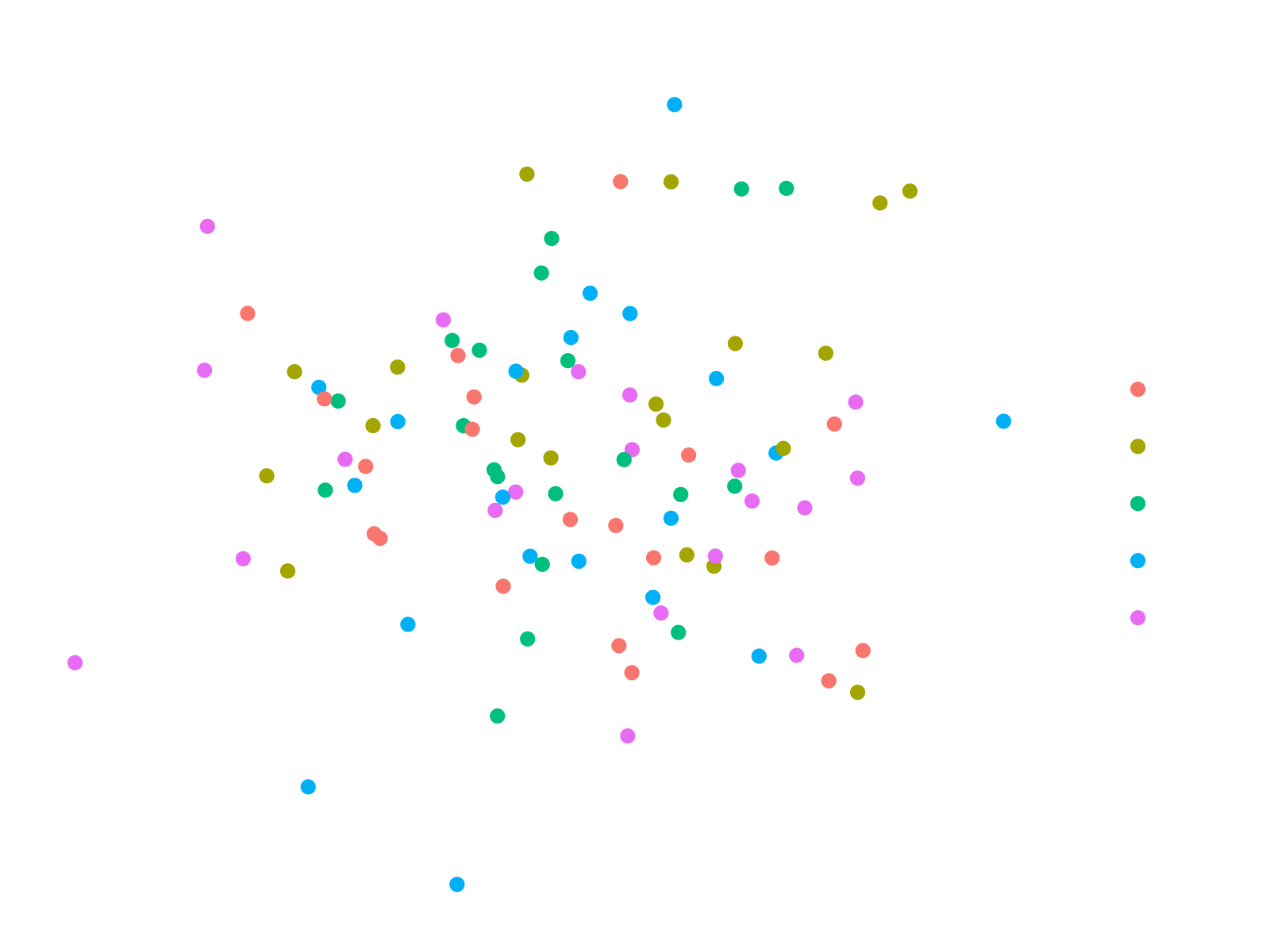```ggp_stata <- ggp + theme_stata() + ggtitle("theme_stata()")
ggp_stata``````ggp_tufte <- ggp + theme_tufte() + ggtitle("theme_tufte()")
ggp_tufte``````ggp_wsj <- ggp + theme_wsj() + ggtitle("theme_wsj()")
ggp_wsj```As you have seen in this section, the ggthemes package can be used to create many different designs, layouts, and graphical styles. In case you want to learn more about the ggthemes package and its source code, you may also have a look at its GitHub page here.

## Video & Further Resources

Do you need further information on the R programming syntax of this article? Then I recommend having a look at the following video on my YouTube channel. I’m explaining the R syntax of this tutorial in the video.

Furthermore, you can find articles on how to plot different kinds of graphics such as xy-plots, density plots, bargraphs, boxplots, histograms, and line plots using the default ggplot2 themes:

In addition, you might have a look at the other RStudio tutorials on this website:

In this R article you have learned how to change the theme of a ggplot2 plot using the ggthemes package. Tell me about it in the comments section below, if you have further questions or comments.

Subscribe to the Statistics Globe Newsletter